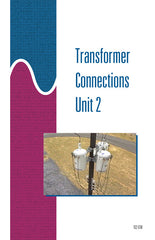Get the Study Guide for this videoFollow the video session-by-session with this handy book. Color illustrations throughout. \$15

Instructor Guide  available

Transformer Connections 2 - DVD

\$ 145.00

Summary

This 56-minute DVD explains how common types of overhead transformers can be connected together. Covers both single-phase and three-phase transformers, with emphasis on three-phase connections of three single-phase transformers. Presents connection theory using phasor diagrams and demonstrates how each of the connections is made. Covers how to make three-phase connections in the following configurations: delta-wye, wye-delta, alternative delta-delta, and alternative wye-delta. Also shows these connections using phasor diagrams. Explains how to connect transformers to form an open bank in delta-delta and wye-delta.

Training Sessions

Introduction – 7 minutes
Describes the basic requirements for connecting units together to form a three-phase bank. Explains connection principles and theory using phasor diagrams. Demonstrates and explains why delta-wye and wye-delta three-phase connections have angular displacements of 30 degrees.

Delta-Wye Connections – 10 minutes
Demonstrates and explains how a delta-wye three-phase connection produces 30 degrees of angular displacement, using subtractive-polarity transformers.

Wye-Delta Connections – 9 minutes
Demonstrates and explains how a three-phase wye-delta connection produces 30 degrees of angular displacement.

Alternative Connections – 12 minutes
Demonstrates and explains: phasor diagrams and angular displacement using alternative connections, how an alternative delta-delta three-phase connection producing 180 degrees of angular displacement can be made, and how an alternative wye-delta three-phase connection producing 210 degrees of angular displacement can be made.

Open Banks – 9 minutes
Demonstrates and explains: an open delta-delta connection producing 0 degrees of angular displacement and an open wye-delta three-phase connection producing 30 degrees of angular displacement.

Additional Three-Phase Connections – 9 minutes
Demonstrates and explains one example of a three-phase transformer.

Item Code: TC2-DVD